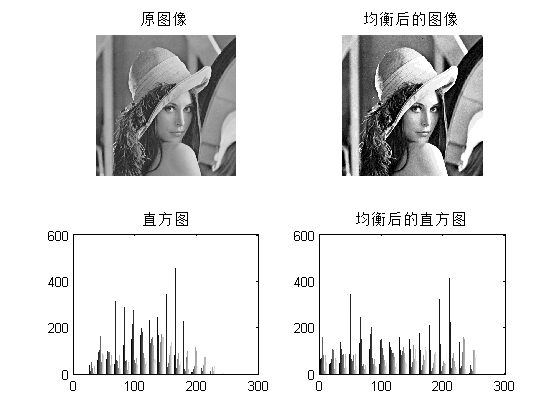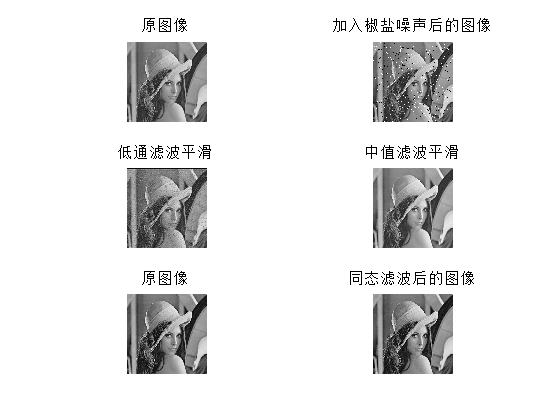# Matlab编程实现图像增强与去噪

Matlab编程实现图像增强与去噪

图像增强使用直方图均衡化方法，利用Matlab的histeq函数实现。使用Matlab自带函数加入椒盐噪声，分别使用低通滤波中值滤波同态滤波进行去噪。下面给出Matlab代码，涉及到的函数如有不清楚的，可以在Matlab命令窗口输入help 函数名或者doc 函数名查看帮助。

使用本程序，请新建一个.m文件，并命名为：imenhden.m，保存，将原始的图片与此m文件都放在Matlab当前工作目录下，然后在命令窗口输入：imenhden(  'lena.png');回车即可，其中lena.png为原始图片名

function [ ] = imenhden( fineName )

%% 读入图像

figure(1);

subplot(2,2,1);

imshow(I);

title('原图像');

%% 直方图均衡化

m = 16;

H = histeq(I,m);

subplot(222);

imshow(H,[]);

title('均衡后的图像');

subplot(223);

hist(double(I),m);

title('直方图');

subplot(224);

hist(double(H),m)

title('均衡后的直方图');

%% 噪声与滤波

figure(2)

subplot(3,2,1);

imshow(I);

title('原图像');

% 加入椒盐噪声并显示

II = imnoise(I,'salt & pepper');

subplot(3,2,2);

imshow(II);

title('加入椒盐噪声后的图像');

% 低通滤波平滑

[B,A] = butter(6,0.2,'low');

J = filter(B,A,double(II));

subplot(3,2,3);

imshow(J,[]);

title('低通滤波平滑');

% 中值滤波平滑

J = medfilt2(II);

subplot(3,2,4);

imshow(J,[]);

title('中值滤波平滑');

% 同态滤波

%I0 = II;M=[];

I1 = log(double(I0)+1);

I2 = fft2(I1);

N=2;D0=0.05*pi;rh=0.8;r=0.5;

[row,col]=size(I2);

for m=1:row

for n=1:col

D1(m,n)=sqrt(m^2+n^2);

H(m,n)=r+(rh/(1+(D0/D1(m,n))^(2*N)));

end

end

I3=I2.*single(H);

I4=ifft2(I3);

I5=exp(I4)-1;

subplot(3,2,5);

imshow(I0,M);

title('原图像');

subplot(3,2,6);

imshow(I5,M);

title('同态滤波后的图像');

end使用如上程序对上图lena图像进行处理，分别得到下图所示的结果：06-2303-04
09-08
01-12
01-067万+
11-191万+
05-231226
05-061万+
10-14
04-111万+
01-23
12-233781
12-17131